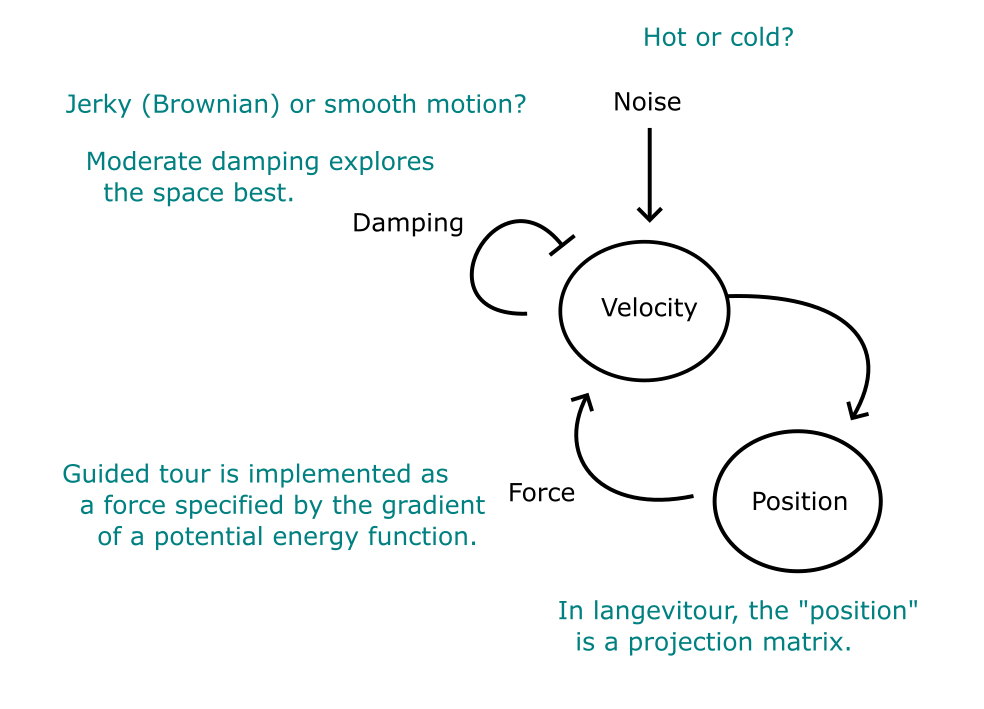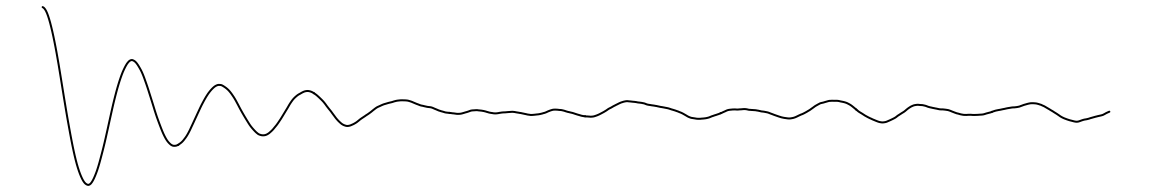palmerpenguins
install.packages("langevitour")
library(langevitour)
langevitour(dataset, labels)

Use directly from R.
or
R Markdown → static HTML.

Interaction, calculations, and drawing done in Javascript.

Seek a “good” projection. (Cook et al., 1995)

Spin randomly. (Asimov, 1985)

## Touring data

GGobi in action

Animate linear, orthonormal projections of data.

Previous software does this by choosing distinct projections then interpolating.

langevitour’s novel feature is using Langevin Dynamics to produce a smooth random path of projections.

• Grand Tour introduced by Daniel Asimov in 1985.

• XGobi, GGobi - interactive desktop applications.

• tourr - R package for tour calculation and animation.

• Ongoing interdisciplinary research by Di Cook and collaborators, e.g. slice tours.

## Langevin Dynamics

“Stochastic Differential Equation”## Constrained Langevin Dynamics

The “position” should always represent an orthonormal projection.

langevitour uses “Position Based Dynamics”, a simple and stable way to maintain constraints in a physics engine.

Each timestep:

1. Update velocity $$\mathbf{v} \leftarrow \mathbf{v} - \mathrm{damping} + \mathrm{noise} + \mathrm{forces}$$
2. Propose new position $$\mathbf{x} \leftarrow \mathbf{x} + \Delta t\ \mathbf{v}$$
3. Fix the proposed $$\mathbf{x}$$ by finding the nearest valid orthonormal projection. (SVD and set singular values to 1)
4. Fix $$\mathbf{v}$$ to be consistent with the actual step taken by $$\mathbf{x}$$.

## Guided Tourlangevitour can sample projections near an optimum defined by a potential energy function.